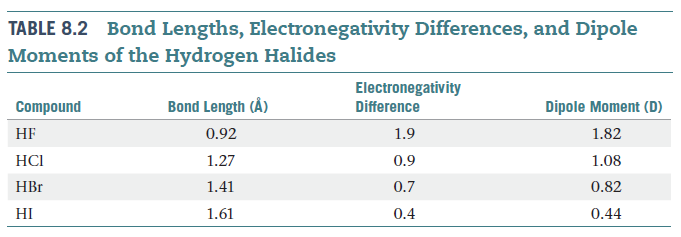Problem: The O-H bond lengths in the water molecule (H2 O) are 0.96 Å, and the H-O-H angle is 104.5°. The dipole moment of the water molecule is 1.85 D.Calculate the magnitude of the bond dipole of the O-H bonds. (Note: You will need to use vector addition to do this.)

FREE Expert Solution

We have to calculate the magnitude of bond dipole for each of the O–H bonds in the H2O (water) molecule.

Bond dipole moment (μ) is the result of charge separation that takes place as a result of bond polarity.

The polarity of a covalent bond is due the difference of electronegativity between the two atoms forming the bond.

To determine the contribution of each covalent bond towards the overall dipole moment, we will follow these steps:

Step 1: Draw a Lewis structure of H2O molecule.

• To draw the Lewis structure, we need to do the following steps:
• Step 1a: Determine the central atom in the molecule.
• Step 1b: Calculate the total number of valence electrons present.
• Step 1c: Draw the Lewis structure for the molecule.

Step 1d: Draw dipole moment arrows.

Step 2: Draw the molecule over an XY axis and determine the equation for individual contributions.

Step 3: Calculate the bond dipole for individual O–H bonds.

89% (200 ratings)
Problem Details
The O-H bond lengths in the water molecule (H2 O) are 0.96 Å, and the H-O-H angle is 104.5°. The dipole moment of the water molecule is 1.85 D.Calculate the magnitude of the bond dipole of the O-H bonds. (Note: You will need to use vector addition to do this.)